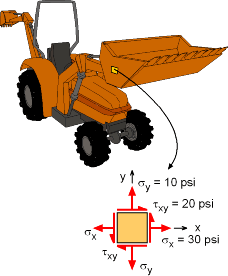Ch 7. Stress Analysis Multimedia Engineering Mechanics PlaneStress PrincipalStresses Mohr's Circlefor Stress Failure PressureVessels
 Chapter 1. Stress/Strain 2. Torsion 3. Beam Shr/Moment 4. Beam Stresses 5. Beam Deflections 6. Beam-Advanced 7. Stress Analysis 8. Strain Analysis 9. Columns Appendix Basic Math Units Basic Equations Sections Material Properties Structural Shapes Beam Equations Search eBooks Dynamics Fluids Math Mechanics Statics Thermodynamics Author(s): Kurt Gramoll ©Kurt GramollMECHANICS - CASE STUDY IntroductionStress State at the Bucket of a Tractor To better understand the stress state for a new tractor, a structural analysis computer program was used to determine stresses in the tractor's front-end bucket. For the location shown, it was determined that the stresses are σx = 30 psi, σy = 10 psi, and τxy = 20 psi. Question Using Mohr's circle, what is 1) the principal direction and principal normal stresses, 2) maximum shear direction and the maximum shearing stress, and 3) the stresses at an angle of 15o? Approach Construct the basic Mohr's circle for the given stress state. Determine the principal direction from the Mohr's circle diagram, and then the principal stresses. Find the maximum shear stress direction from the Mohr's circle diagram. Rotate the stress element 15o using Mohr's circle.

Practice Homework and Test problems now available in the 'Eng Mechanics' mobile app
Includes over 400 problems with complete detailed solutions.
Available now at the Google Play Store and Apple App Store.